# Complex Number & Matrix Calc

100+Everyone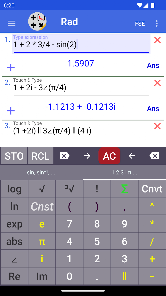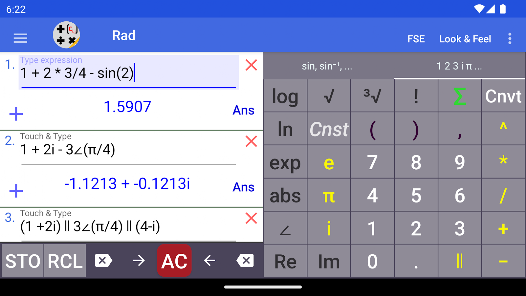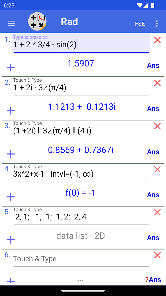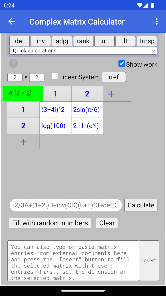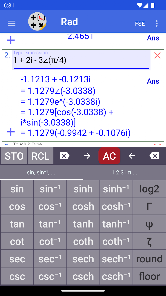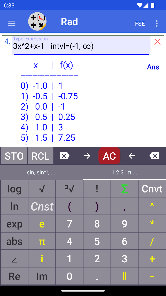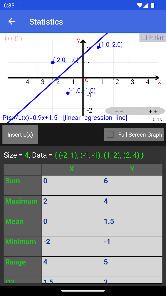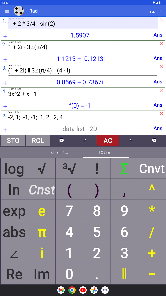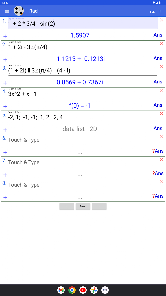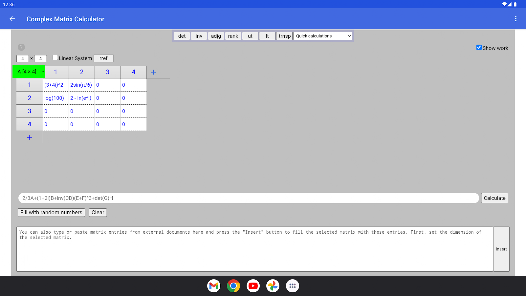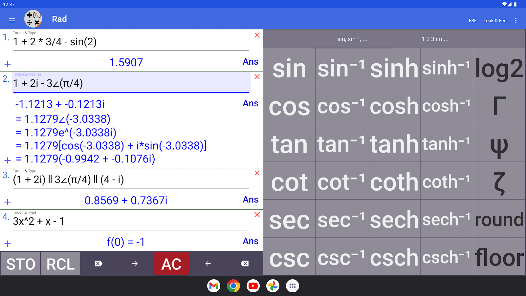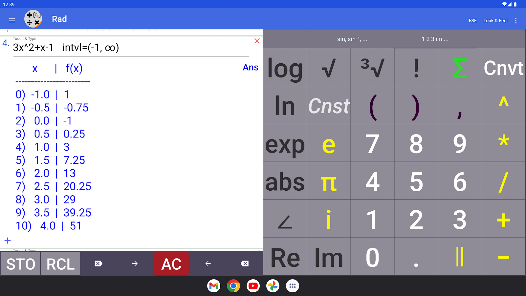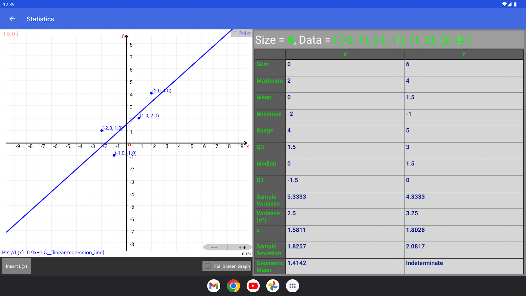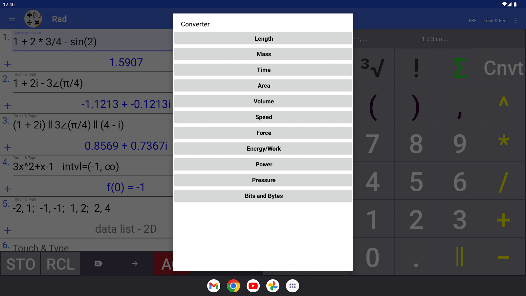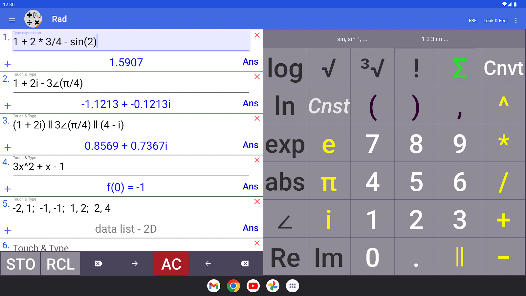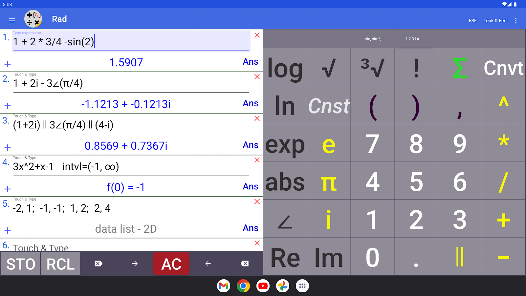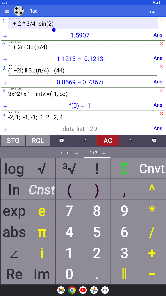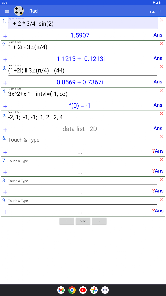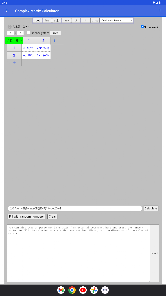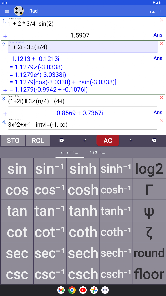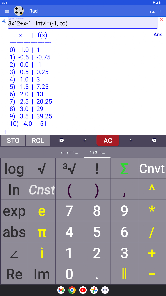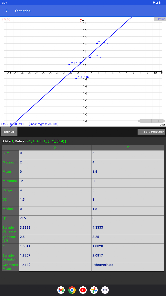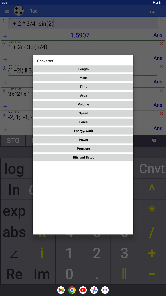Complex number calculator, complex matrix calculator, a fully developed scientific calculator for calculations of real, imaginary and, in general, complex numbers and matrix expressions. Also for solving systems of linear equations by performing Gauss-Jordan eliminations on augmented matrices.

You can use all standard mathematical functions & operations and more:

• Addition, subtraction, multiplication, division, parallel summation
• Power, exponential & logarithmic functions
• Trigonometric functions and their Inverses
• Hyperbolic functions and their Inverses
• Gamma function, Γ
• Psi function, Ψ
• Zeta function, ζ

Matrices & linear Systems
• Perform matrix algebra.
• Accepts any size matrix
• Determinant, inverse, rank, adjugate, reduced row echelon form, triangular forms
• Augmented matrix
• Solve linear systems
• Shows work.

With it's unique and intuitive user interface, this scientific/complex number calculator computes mathematical expressions containing complex numbers in standard (rectangular) a+bi and polar (phasor) r∠(θ) forms and optionally converts the results to rectangular (standard), polar and other modular forms.

(long press i or ∠ to see all forms of the calculated result).

Scientific/Complex number calculations can be performed in RAD (radians) or DEG (degrees) mode.

The results can be displayed with fixed, scientific and engineering notations.

Other Features

• You can also enter functions f(x) or parametric equations p(t) and generate table of values.

Do statistics with one or two dimensional real data (you can also use expressions of complex numbers for data as long as they evaluate to real numbers):
• x1, x2, …
• x1, y1; x2, y2; …
Press Σ to do the calculations.

• Calculate combinations C(n, r) and permutations P(n, r).

• Scientific constants.
• Unit converter (Mass, Length, Velocity, ...)

This scientific calculator / complex number calculator / complex matrix calculator contains full built-in instructions.
Updated on
Nov 29, 2022

## Data safety

Safety starts with understanding how developers collect and share your data. Data privacy and security practices may vary based on your use, region, and age. The developer provided this information and may update it over time.No data shared with third parties